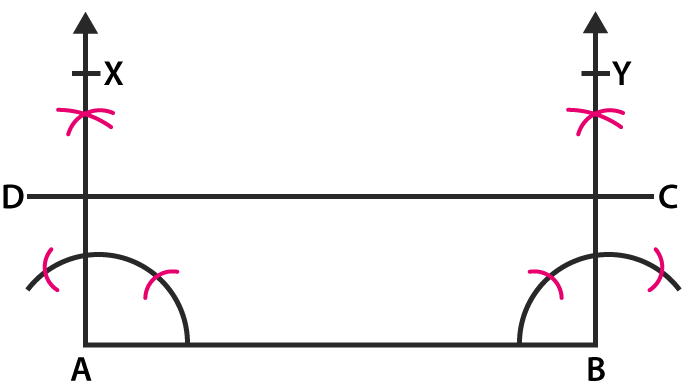# RD Sharma Solutions for Class 6 Maths Chapter 19: Geometrical Constructions Exercise 19.6

In this exercise, students learn how to construct angles with the help of the ruler and compasses. The solutions for the exercise-wise problems are designed by faculty at BYJU’S based on the latest CBSE guidelines. The students can use the solutions in order to understand other methods which can be used to solve the complex problems in a shorter duration. To improve time management which is an important aspect from the exam point of view, students can download RD Sharma Solutions Class 6 Maths Chapter 19 Geometrical Constructions Exercise 19.6 PDF which are available here.

## RD Sharma Solutions for Class 6 Maths Chapter 19: Geometrical Constructions Exercise 19.6 Download PDF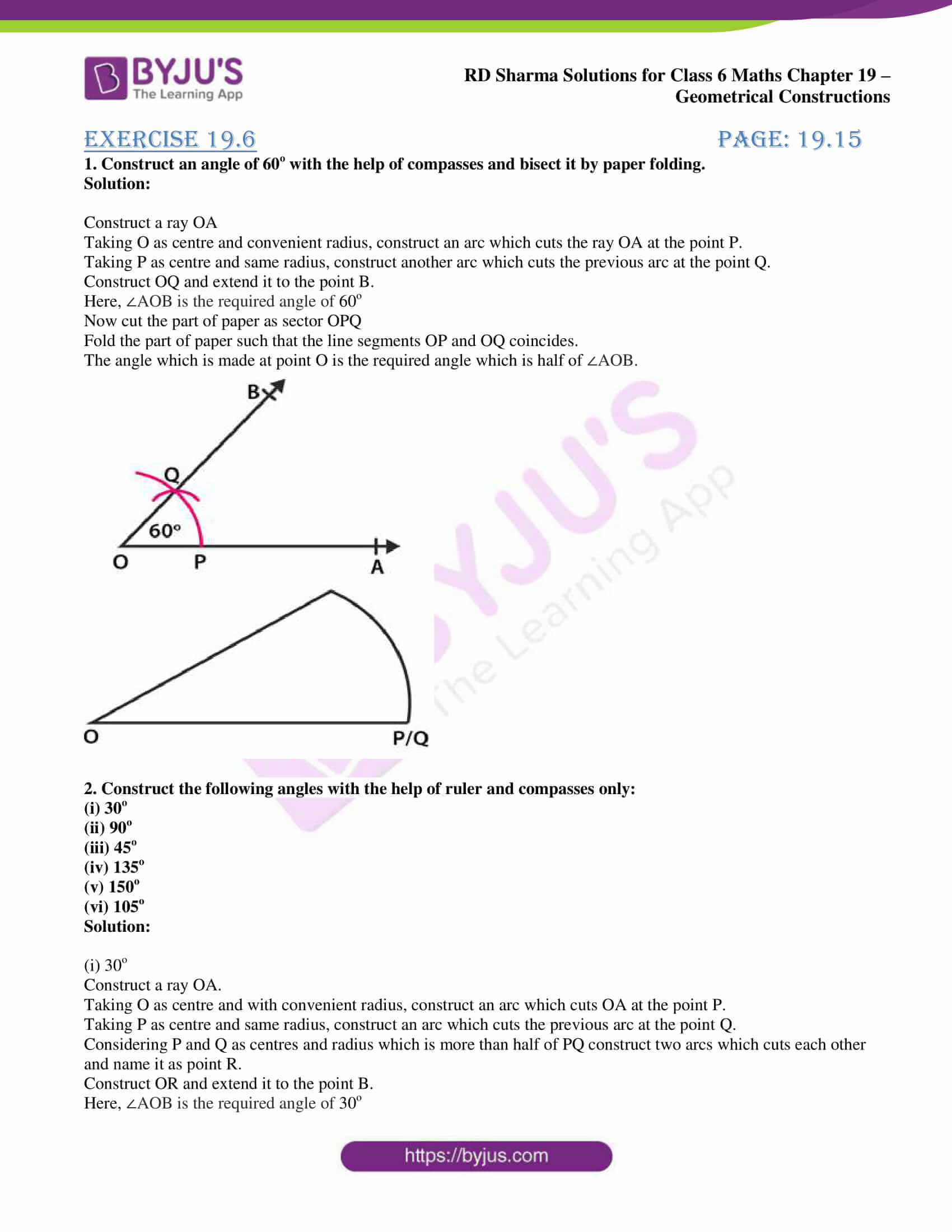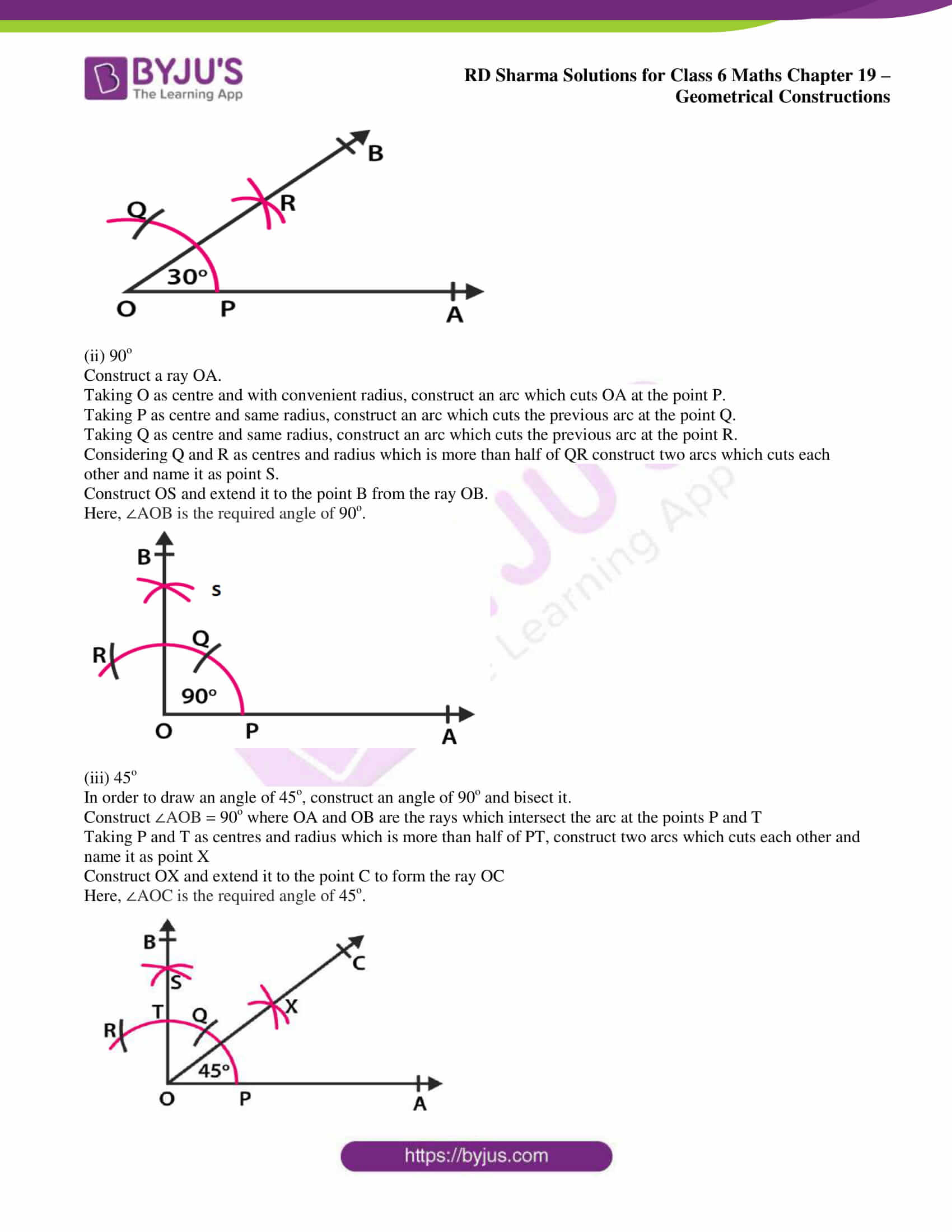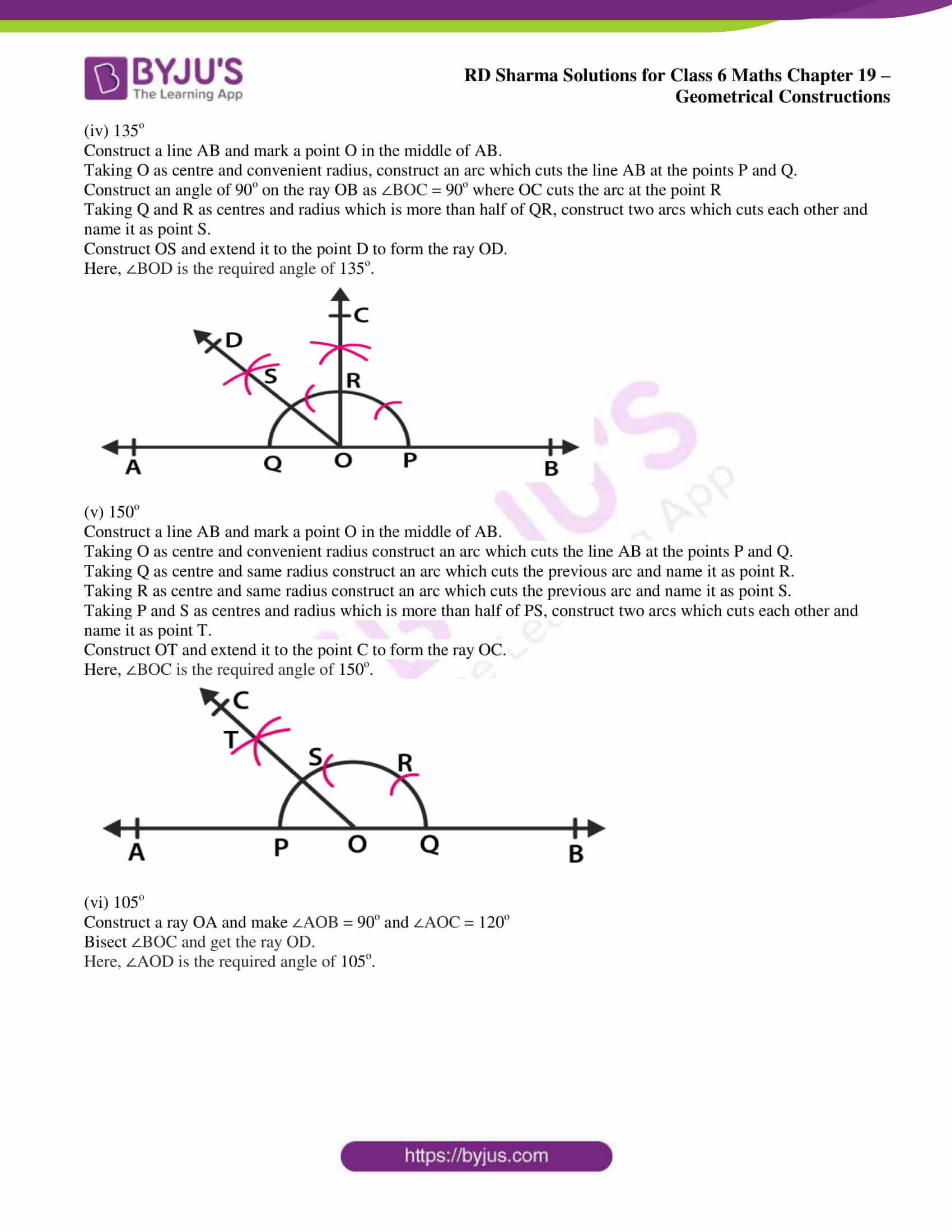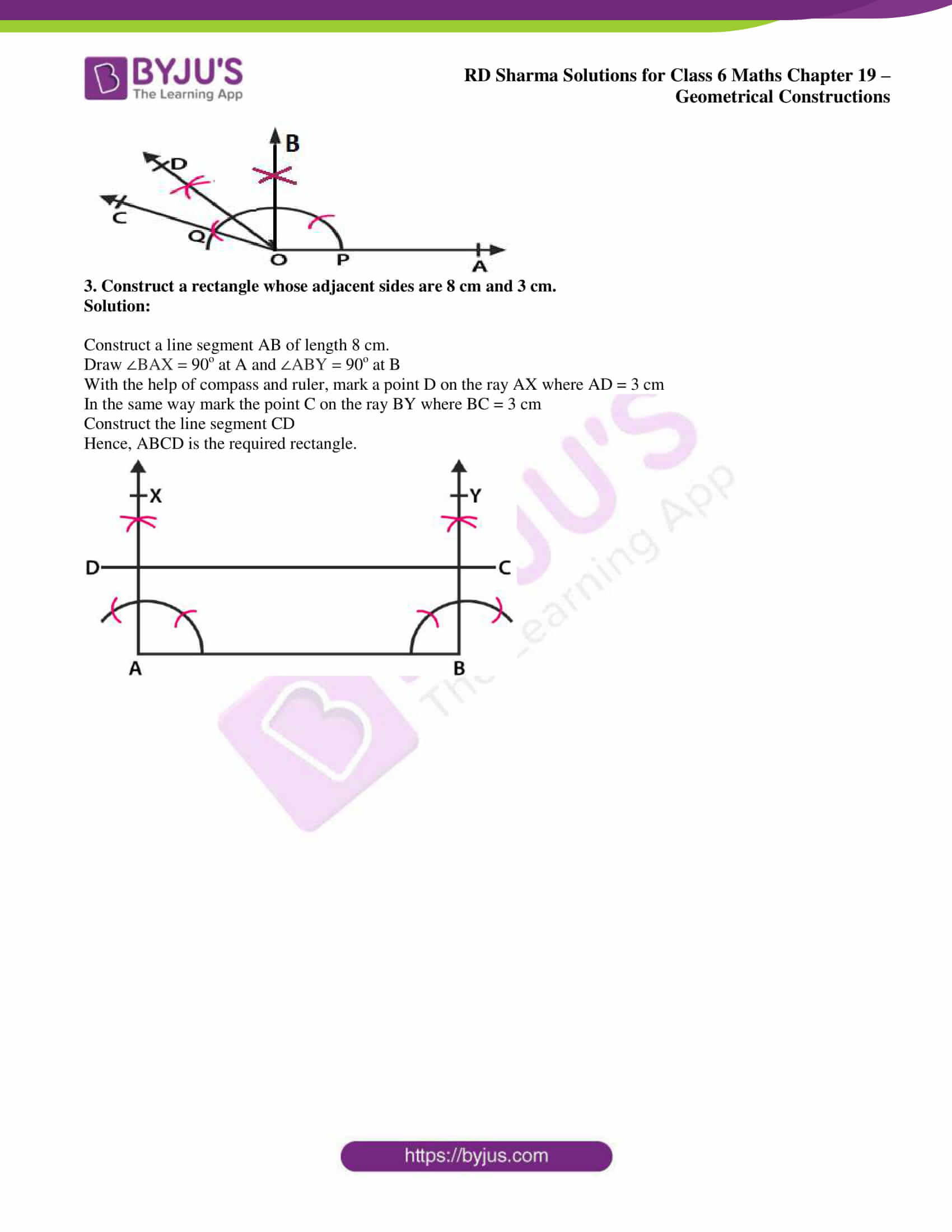### Exercise 19.6 page: 19.15

1. Construct an angle of 60o with the help of compasses and bisect it by paper folding.

Solution:

Construct a ray OA

Taking O as centre and convenient radius, construct an arc which cuts the ray OA at the point P.

Taking P as centre and same radius, construct another arc which cuts the previous arc at the point Q.

Construct OQ and extend it to the point B.

Here, ∠AOB is the required angle of 60o

Now cut the part of paper as sector OPQ

Fold the part of paper such that the line segments OP and OQ coincides.

The angle which is made at point O is the required angle which is half of ∠AOB.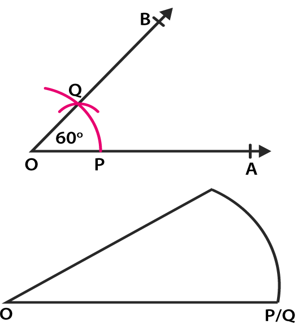2. Construct the following angles with the help of ruler and compasses only:

(i) 30o

(ii) 90o

(iii) 45o

(iv) 135o

(v) 150o

(vi) 105o

Solution:

(i) 30o

Construct a ray OA.

Taking O as centre and with convenient radius, construct an arc which cuts OA at the point P.

Taking P as centre and same radius, construct an arc which cuts the previous arc at the point Q.

Considering P and Q as centres and radius which is more than half of PQ construct two arcs which cuts each other and name it as point R.

Construct OR and extend it to the point B.

Here, ∠AOB is the required angle of 30o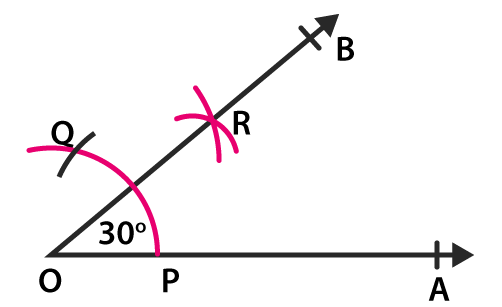(ii) 90o

Construct a ray OA.

Taking O as centre and with convenient radius, construct an arc which cuts OA at the point P.

Taking P as centre and same radius, construct an arc which cuts the previous arc at the point Q.

Taking Q as centre and same radius, construct an arc which cuts the previous arc at the point R.

Considering Q and R as centres and radius which is more than half of QR construct two arcs which cuts each other and name it as point S.

Construct OS and extend it to the point B from the ray OB.

Here, ∠AOB is the required angle of 90o.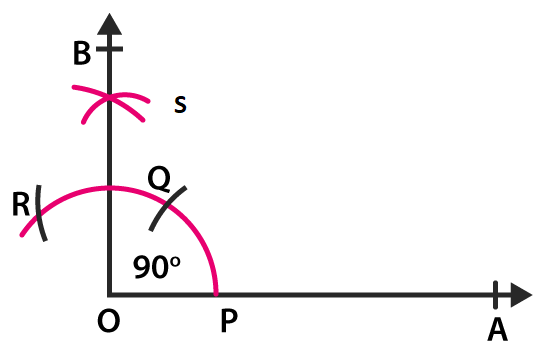(iii) 45o

In order to draw an angle of 45o, construct an angle of 90o and bisect it.

Construct ∠AOB = 90o where OA and OB are the rays which intersect the arc at the points P and T

Taking P and T as centres and radius which is more than half of PT, construct two arcs which cuts each other and name it as point X

Construct OX and extend it to the point C to form the ray OC

Here, ∠AOC is the required angle of 45o.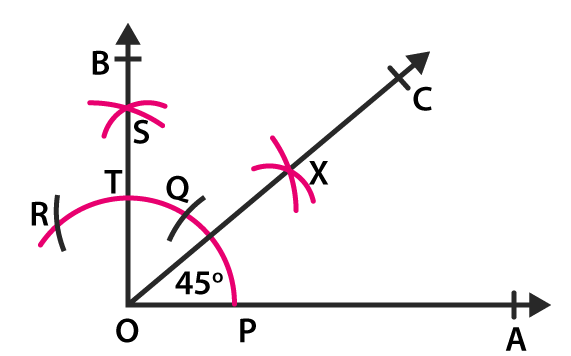(iv) 135o

Construct a line AB and mark a point O in the middle of AB.

Taking O as centre and convenient radius, construct an arc which cuts the line AB at the points P and Q.

Construct an angle of 90o on the ray OB as ∠BOC = 90o where OC cuts the arc at the point R

Taking Q and R as centres and radius which is more than half of QR, construct two arcs which cuts each other and name it as point S.

Construct OS and extend it to the point D to form the ray OD.

Here, ∠BOD is the required angle of 135o.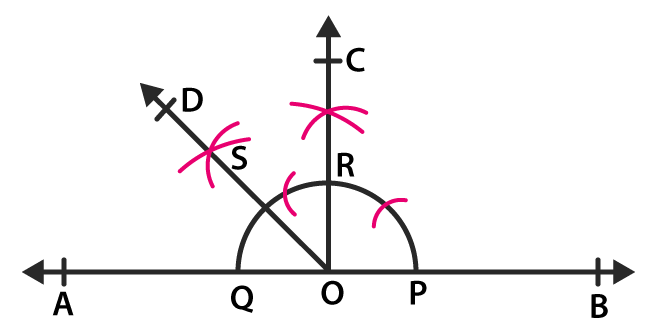(v) 150o

Construct a line AB and mark a point O in the middle of AB.

Taking O as centre and convenient radius construct an arc which cuts the line AB at the points P and Q.

Taking Q as centre and same radius construct an arc which cuts the previous arc and name it as point R.

Taking R as centre and same radius construct an arc which cuts the previous arc and name it as point S.

Taking P and S as centres and radius which is more than half of PS, construct two arcs which cuts each other and name it as point T.

Construct OT and extend it to the point C to form the ray OC.

Here, ∠BOC is the required angle of 150o.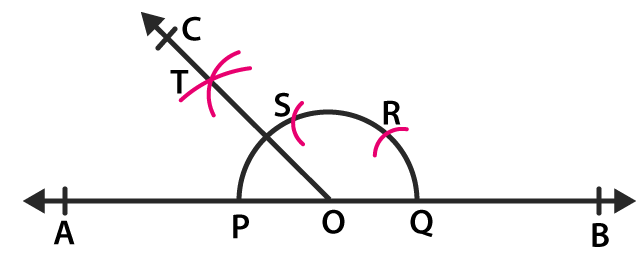(vi) 105o

Construct a ray OA and make ∠AOB = 90o and ∠AOC = 120o

Bisect ∠BOC and get the ray OD.

Here, ∠AOD is the required angle of 105o.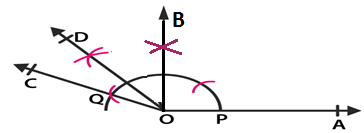3. Construct a rectangle whose adjacent sides are 8 cm and 3 cm.

Solution:

Construct a line segment AB of length 8 cm.

Draw ∠BAX = 90o at A and ∠ABY = 90o at B

With the help of compass and ruler, mark a point D on the ray AX where AD = 3 cm

In the same way mark the point C on the ray BY where BC = 3 cm

Construct the line segment CD

Hence, ABCD is the required rectangle.